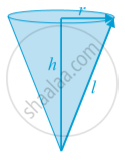# Surface Area of a Right Circular Cone

#### notes

Now the area of each triangle = 1/2 × base of each triangle ×  l
But the curved portion of the figure makes up the perimeter of the base of the cone and the circumference of the base of the cone = 2πr, where r is the base radius of the cone.
So,
Curved Surface Area of a Cone = 1/2 × l × 2πr  = πrl

where r is its base radius and l its slant height.
Note that l^2 = r^2 + h^2 (as can be seen from in following fig. by applying Pythagoras Theorem. Here h is the height of the cone.Therefore, l = sqrt (r^2 + h^2)
Now if the base of the cone is to be closed, then a circular piece of paper of radius r is also required whose area is πr^2.
So,
Total Surface Area of a Cone = πrl + πr^2 = πr(l + r)

If you would like to contribute notes or other learning material, please submit them using the button below.

### Shaalaa.com

Surface Area of a Right Circular Cone [00:04:32]
S
0%